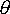QUESTION #184

# Why does dispersion take place when light is passed through prism and not through glass slab?

A light ray is refracted (bent) when it passes from one medium to another at an angle and its speed changes. At the interface, it is bent in one direction if the material it enters is denser (when light slows down) and in the OTHER direction if the material is less dense (when light speeds up). Because different wavelengths (colors) of light travel through a medium at different speeds, the amount of bending is different for different wavelengths. Violet is bent the most and red the least because violet light has a shorter wavelength, and short wavelengths travel more slowly through a medium than longer ones do. Because white light is made up of ALL visible wavelengths, its colors can be separated (dispersed) by this difference in behavior.

When light passes through glass, it encounters TWO interfaces--one entering and the other leaving. It slows down at the first interface and speeds back up at the second. If the two interface surfaces are parallel to each other, as in a 'slab' of glass, all of the bending (and dispersion) that takes place at the first interfaces is exactly reversed at the second, 'undoing' the effect of the first interface; so although the emerging ray of light is displaced slightly from the entering ray, it travels in the same direction as the incoming ray and all wavelengths that separated at the first interface are re-combined.

If the second interface is NOT parallel to the first, as in a prism, the effects of the first interface are NOT reversed and the colors separated at that interface continue along different paths upon leaving the glass.
Answered by: Paul Walorski, B.A. Physics, Part-time Physics Instructor

Well, in fact, dispersion does occur when light is passed through a glass slab - it is just harder to observe that way. Lets summarize how this happens:

Refraction of light when it passes from one medium to the other obeys Snell's law, which states:

n1 sin(1) = n2 sin (2)

Where n1 and n2 are the indices of refraction of the two media, and1 and2 is the angle the ray of light makes with the normal to the surface in the two media. The index of refraction for air is (almost) 1, while for glass it is about 1.5 (or so). So, when light enters a medium with higher index of refraction, it will be bent toward the normal, i.e. end up closer to being perpendicular to the surface.

So far so good. But how does that explain the dispersion of different colors of light? The answer lies in n, the index of refraction. The index of refraction is not a constant, but rather varies with the frequency (hence, wavelength) of the light involved. So, different colors are bent by different amounts. So, in a prism, light goes through two surfaces, which are not parallel and as a result, every color exiting the prism travels in a different direction - splitting up clearly over a short distance.

Now, for a slab the situation is different. Since the two surfaces are parallel, the direction of any color light is not changed by going through the slab - it is only offset by a small amount (that's exactly why objects look displaced a little when looked at through a glass slab). This offset depends on the angle of incidence, the index of refraction, and is directly proportional to the thickness of the slab. As a result, every color is offset by a very small amount with respect to each other, and the color separation is only observable at the edges, or if one uses a very small spot of light, and a very thick slab of glass.
Answered by: Yasar Safkan, B.S. Physics, Ph.D. Candidate, MIT